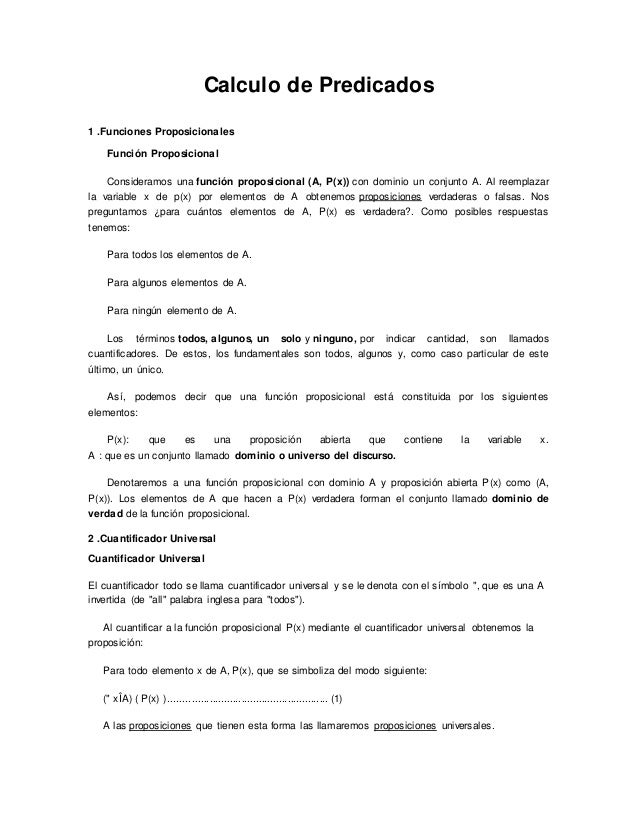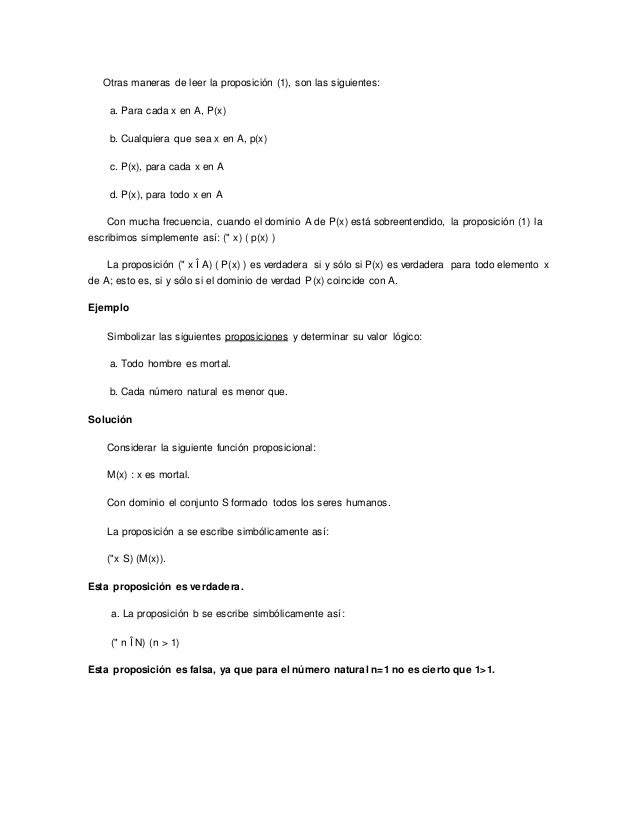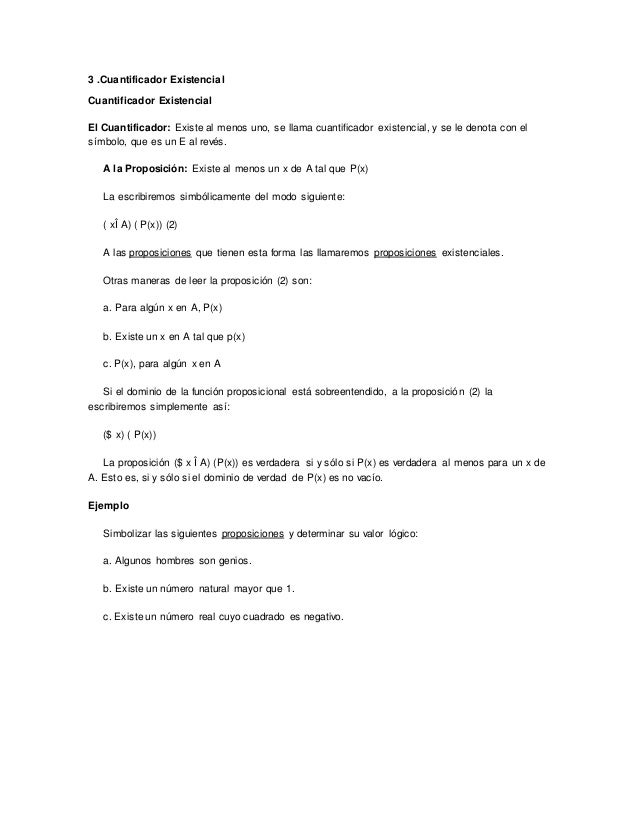Successfully reported this slideshow.Upcoming SlideShare
×

of0

Share

función proporcional y sus respectivos elementos que lo conforman.
reglas de la negación de cuantificadores.

See all

### Related Audiobooks

#### Free with a 30 day trial from Scribd

See all
• Be the first to like this

2. 2. Otras maneras de leer la proposición (1), son las siguientes: a. Para cada x en A, P(x) b. Cualquiera que sea x en A, p(x) c. P(x), para cada x en A d. P(x), para todo x en A Con mucha frecuencia, cuando el dominio A de P(x) está sobreentendido, la proposición (1) la escribimos simplemente así: (" x) ( p(x) ) La proposición (" x Î A) ( P(x) ) es verdadera si y sólo si P(x) es verdadera para todo elemento x de A; esto es, si y sólo si el dominio de verdad P(x) coincide con A. Ejemplo Simbolizar las siguientes proposiciones y determinar su valor lógico: a. Todo hombre es mortal. b. Cada número natural es menor que. Solución Considerar la siguiente función proposicional: M(x) : x es mortal. Con dominio el conjunto S formado todos los seres humanos. La proposición a se escribe simbólicamente así: ("x S) (M(x)). Esta proposición es verdadera. a. La proposición b se escribe simbólicamente así: (" n Î N) (n > 1) Esta proposición es falsa, ya que para el número natural n=1 no es cierto que 1>1.
3. 3. 3 .Cuantificador Existencial Cuantificador Existencial El Cuantificador: Existe al menos uno, se llama cuantificador existencial, y se le denota con el símbolo, que es un E al revés. A la Proposición: Existe al menos un x de A tal que P(x) La escribiremos simbólicamente del modo siguiente: ( xÎ A) ( P(x)) (2) A las proposiciones que tienen esta forma las llamaremos proposiciones existenciales. Otras maneras de leer la proposición (2) son: a. Para algún x en A, P(x) b. Existe un x en A tal que p(x) c. P(x), para algún x en A Si el dominio de la función proposicional está sobreentendido, a la proposición (2) la escribiremos simplemente así: (\$ x) ( P(x)) La proposición (\$ x Î A) (P(x)) es verdadera si y sólo si P(x) es verdadera al menos para un x de A. Esto es, si y sólo si el dominio de verdad de P(x) es no vacío. Ejemplo Simbolizar las siguientes proposiciones y determinar su valor lógico: a. Algunos hombres son genios. b. Existe un número natural mayor que 1. c. Existe un número real cuyo cuadrado es negativo.
6. 6. Solución a. ~ [(\$ n Î N) (n2 = n )] º (" n Î N) ~ ( n2 = n) (Negación de cuantificadores) º (" n Î N) ( n2 ¹ n) (Negación de la función proposicional) b. ~ [(" x Î R) (x > 2 ® x2 > 3)] º (\$ x Î R) ~ (x > 2 ® x2 > 3) º (\$ x Î R) ~ (~ (x > 2) Ú (x2 >3) (L. del condicional) º (\$ x Î R) (x > 2) Ù (x2 £ 3) (L.de De Morgan) Proposiciones con dos Cuantificadores Podemos considerar funciones proposicionales de varias variables de la forma (A,B,C,P(x,y,z)), pero en nuestro caso trabajaremos con funciones proposicionales de dos variables, las cuales denotaremos por (A,B,P(x)) con dominio de x el conjunto A y dominio de y el conjunto B. Así podemos obtener las siguientes proposiciones: (" xÎ A)(" yÎ B)(P(x,y))º (" yÎ B)(" xÎ A)(P(x,y)) 1. (\$ xÎ A)(\$ yÎ B)(P(x,y)) º (\$ yÎ B)(\$ xÎ A)(P(x,y)) 2. (" xÎ A)(\$ yÎ B)(P(x,y)) 3. (" yÎ B)(\$ xÎ A)(P(x,y)) 4. (\$ xÎ A)(" yÎ B)(P(x,y)) 5. (\$ yÎ B)(" xÎ A)(P(x,y)) Proposiciones como las anteriores son llamadas funciones proposicionales de dos variables. De dichas proposiciones obtenemos el valor lógico, analizando el dominio de sus variables y los cuantificadores que contiene. Ejemplo Determinar el valor lógico de las siguientes proposiciones: 1. (" xÎ N)(\$ yÎ N) (y> x) 2. (\$ xÎ R)(" yÎ R)(x+y = 0) 3. (" xÎ R)(\$ yÎ R)(x+y = 0)
7. 7. Solución VL[(" xÎ N)(\$ yÎ N)(y> x)] = 1, ya que para cualquier x en N existe y = x+1 tal que y> x. VL[(\$ xÎ R)(" yÎ R)(x+y = 0)] = 0, no existe ningún número real que sumado con todo número real sea igual a cero. VL[(" xÎ R)(\$ yÎ R)(x+y = 0)] = 0, ya que dado un número real x existe y = -x tal que x+y=0. Veamos ahora como podemos negar proposiciones con dos cuantificadores. Negación de Proposiciones con dos Cuantificadores ~ [(" xÎ A)(\$ yÎ B)(P(x,y))] º (\$ xÎ A)(" yÎ B)(~ P(x,y)) ~ [(" xÎ A)(" yÎ B)(P(x,y))] º (\$ xÎ A)(\$ yÎ B)(~ P(x,y)) ~ [(\$ xÎ A)(" yÎ B)(P(x,y))] º (" xÎ A)(\$ yÎ B)(~ P(x,y)) ~ [(\$ yÎ B)(\$ xÎ A)(P(x,y))] º (" yÎ B)(" xÎ A)(~ P(x,y)) Ejemplo Negar la proposición (\$ xÎ R)(" yÎ R)(x+y = 0) Solución ~ [(\$ xÎ R)(" yÎ R)(x+y = 0)] º (" xÎ R)(\$ yÎ R)(~ (x+y = 0)) º (" xÎ R)(\$ yÎ R)(x+y ¹ 0))

Total views

239

On Slideshare

0

From embeds

0

Number of embeds

5

2

Shares

0